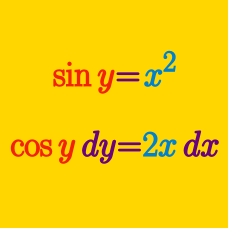Calculus

# Implicit Differentiation - Radical Functions

If $$\sqrt{x^2}+\sqrt{y^2}=4,$$ what is $$\displaystyle \frac{dy}{dx}?$$

If $$x=y\sqrt{11+y}$$, what is $$\displaystyle \frac{dy}{dx}?$$

Find $$\displaystyle \frac{dy}{dx}$$ for $$7\sqrt{x}+6\sqrt{y}=13y^2$$ at the point $$(1,1).$$

Given $f(x)=\sqrt{\frac{x}{x^2+124}},$ what is the value of $$f'(1)?$$

If $$\sqrt{3x}+\sqrt{y}=\sqrt{5}$$, what is $$\displaystyle \frac{dy}{dx}?$$

×